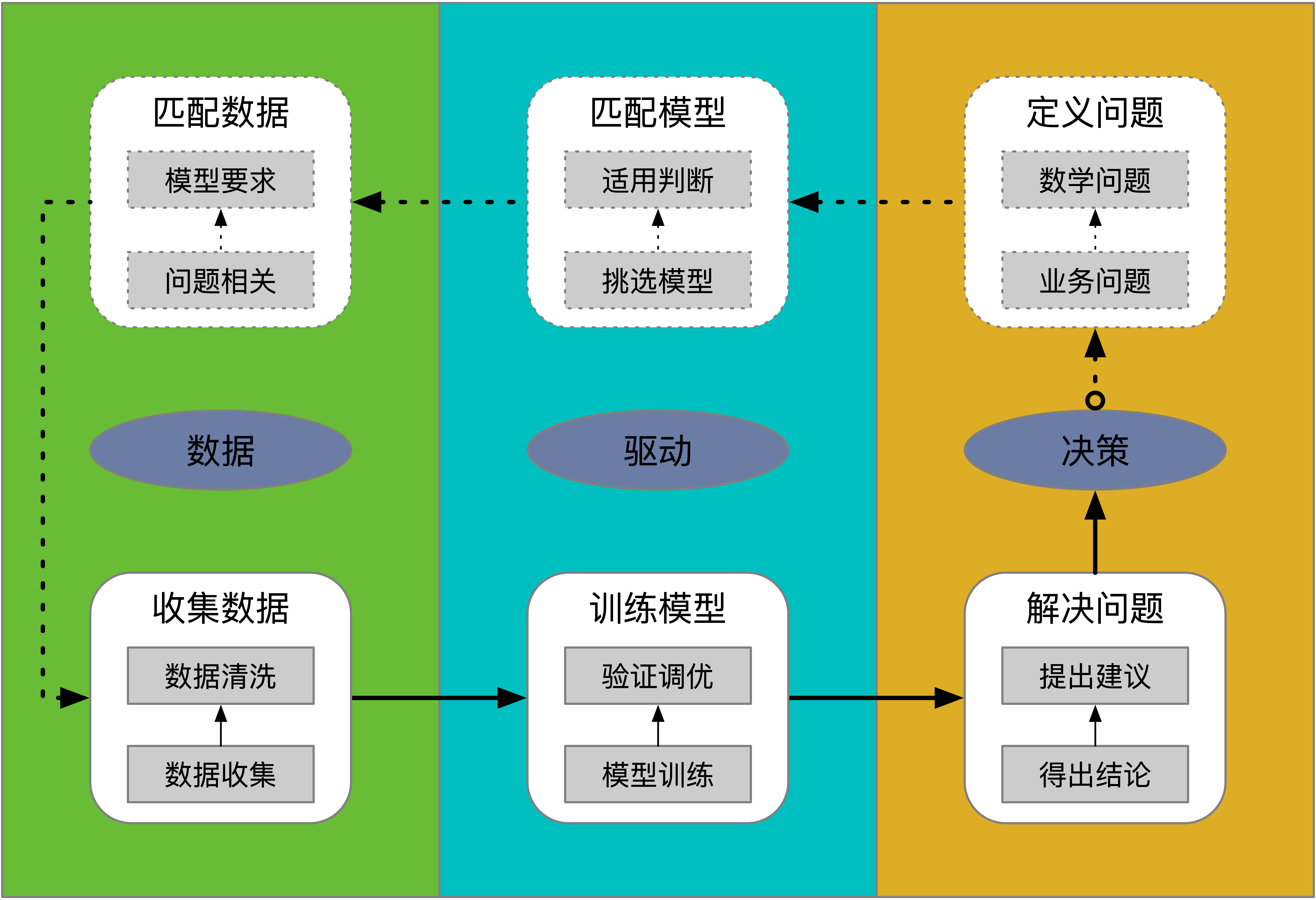1. 数据：指的是数据的收集和整理，典型的就是建立数仓的过程；
2. 驱动：指的是驱动的方法和过程，典型的包括统计描述、统计推断、统计建模等；
3. 决策：指的是决策的场景和问题，典型的包括用户增长、风险控制等；• 数据科学
• 数据
• 数据仓库
• 数仓存储
• HDFS
• HIVE
• HBase
• Mysql
• Impala
• ClickHouse
• 数仓ETL
• 离线计算
• MapReduce
• Spark
• 实时计算
• Spark Streaming
• 数仓模型
• 数仓规范
• 维度建模
• 数仓编程
• SQL
• Python
• Scala
• R
• 数据治理
• 元数据管理
• 标准管理
• 质量管理
• 安全管理
• 共享管理
• 驱动
• 统计描述
• 维度-指标聚合
• 统计推断
• AB 实验
• 假设检验
• 统计建模
• 相关分析
• 相关性
• 预测分析
• 机器学习
• 因果分析
• 因果推断
• 决策
• 通用知识
• 用户增长
• 风险控制
• 领域知识
• 游戏
• 电商
• 社交
• 金融
• 医疗
• 问题导向
• 发现问题
• 总结过去
• 过去发生了什么？
• 指标描述
• 趋势分析
• 监控当下
• 当前正在发生什么？
• 异常监控
• 异动归因
• 预测未来
• 未来可能会发生什么？
• 分类
• 回归
• 聚类
• 解决问题
• 选择
• 为了…应该选择哪种方案?
• 开放
• 为了…应该怎么做?